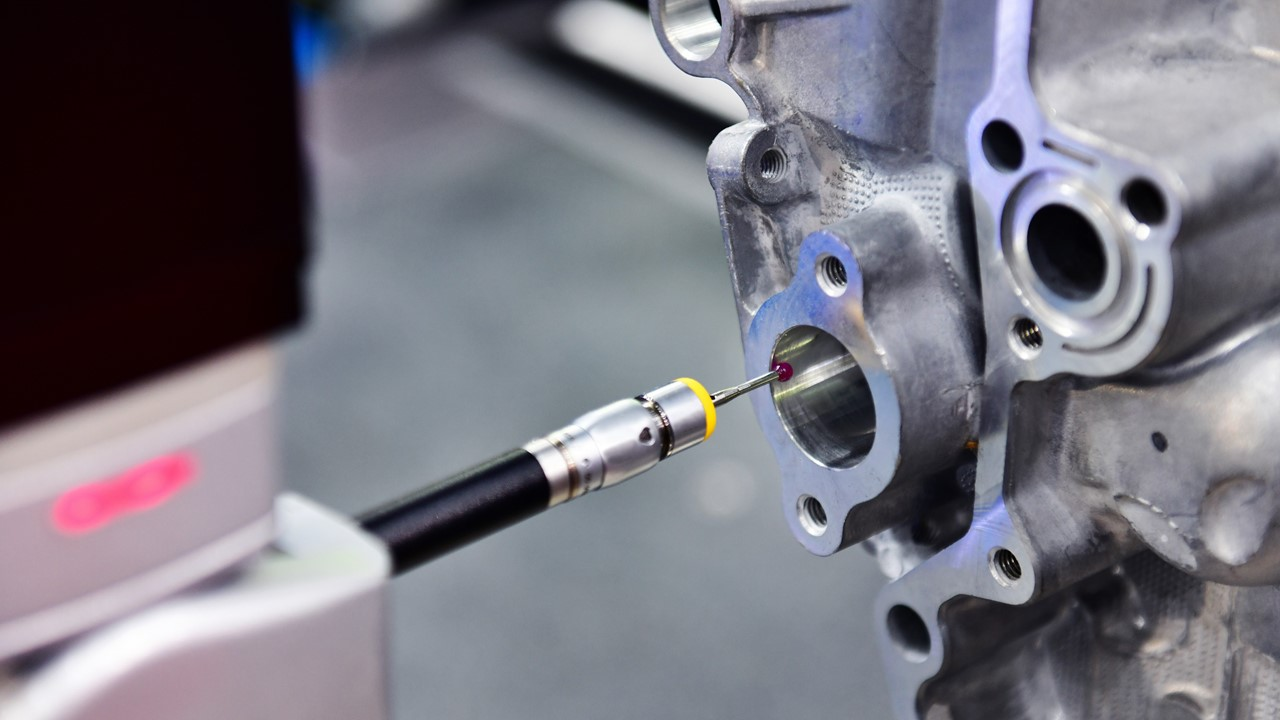# How neural networks operate

Neural networks are computational models inspired by the structure and function of the human brain. They are composed of interconnected artificial neurons (also known as nodes or units) organized in layers. These networks learn from data by adjusting the weights and biases associated with the connections between neurons.

Here’s a high-level overview of how neural networks operate:

• Input Layer: The neural network begins with an input layer that receives the raw data or features. Each neuron in the input layer represents a feature or attribute of the data.
• Hidden Layers: After the input layer, one or more hidden layers can be present in the network. Hidden layers are composed of neurons that receive input from the previous layer and apply a mathematical transformation to produce an output. Hidden layers enable the network to learn complex patterns and relationships in the data.
• Weights and Biases: Each connection between neurons in adjacent layers has an associated weight and bias. The weights determine the strength of the connection, while the biases introduce an offset. Initially, these weights and biases are assigned randomly.
• Activation Function: Neurons in the hidden layers and output layer typically apply an activation function to the weighted sum of their inputs plus the bias. The activation function introduces non-linearity into the network, enabling it to learn and model complex relationships.
• Forward Propagation: During the forward propagation phase, the neural network computes an output based on the input data. The outputs are calculated by propagating the inputs through the layers, applying the activation functions, and using the current weights and biases.
• Loss Function: The output of the neural network is compared to the desired output using a loss function. The loss function quantifies the difference between the predicted output and the actual output. The goal of training is to minimize this loss.• Backpropagation: Backpropagation is the process of adjusting the weights and biases of the network based on the computed loss. It works by calculating the gradient of the loss function with respect to the weights and biases, and then updating them in the direction that reduces the loss. This process is typically done using optimization algorithms like gradient descent.
• Training: The network goes through multiple iterations of forward propagation and backpropagation to update the weights and biases, gradually reducing the loss. This iterative process is known as training. The training is typically performed on a labeled dataset, where the desired outputs are known, allowing the network to learn from the provided examples.
• Prediction: Once the neural network has been trained, it can be used for making predictions on new, unseen data. The forward propagation process is applied to the new input data, and the network produces an output based on the learned weights and biases.
• Evaluation and Iteration: The performance of the trained neural network is evaluated using various metrics and validation datasets. If the performance is not satisfactory, the network can be adjusted by modifying the architecture, tuning hyperparameters, or acquiring more training data. This iterative process continues until the desired performance is achieved.

It’s important to note that this is a simplified explanation of neural networks, and there are many variations and additional concepts involved in different types of neural networks, such as convolutional neural networks (CNNs) for image processing or recurrent neural networks (RNNs) for sequential data.

##### Related Articles##### Floundering teams – A source of failure for Six Sigma Projects##### What is Industry 4.0 and How will it transform our industry?##### What is Y-Shaped Matrix Diagram?